# Act 10 Act 9 Act 8 Act 7

• Slides: 28Act 10 Act 9 Act 8 Act 7 Act 6 Act 5 Act 4 Act 3 Act 2 Act 1 Index How to make a clinometerIndex Act 1 Act 3 Act 4 Student Activity 5: Act 5 Student Activity 6: Student Activity 7: Student Activity 8: Act 10 Act 9 Act 8 Student Activity 4: Act 6 Student Activity 1: Student Activity 2: Student Activity 3 : Act 7 Act 2 How to make a clinometer Student Activity 9: : Student Activity 10: Index Me and my shadow Labelling Sides in Right Angled Triangles Calculating ratios for similar right angled triangles with angles of 30° Calculating ratios for similar right angled triangles with angles of 45° Calculating ratios for similar right angled triangles with angles of 50° Calculating ratios for similar right angled triangles with angles of 60° Calculating ratios for similar right angled triangles with angles of 70° Master table of class results for ratios of sides in right angled triangles Using the master table of class results answer the following questions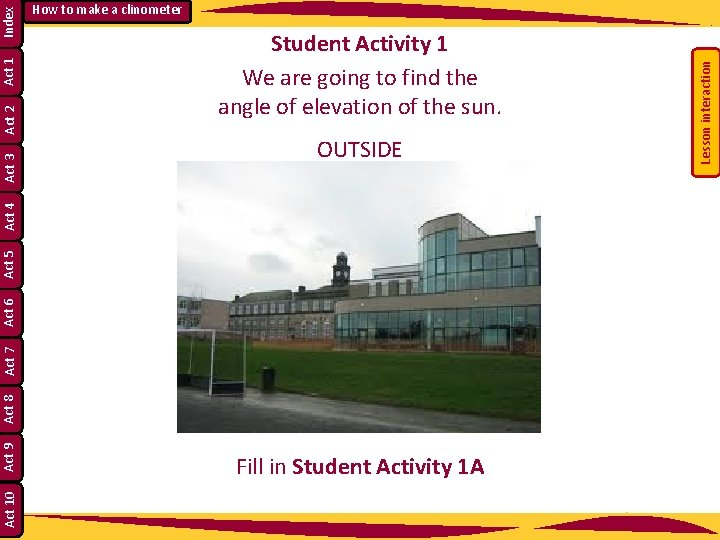OUTSIDE Act 5 Act 6 Act 7 Act 8 Act 9 Act 10 Fill in Student Activity 1 A Lesson interaction Index Act 1 Act 2 Student Activity 1 We are going to find the angle of elevation of the sun. Act 4 Act 3 How to make a clinometerAct 10 Act 9 Act 8 Act 7 Act 6 Act 5 Act 4 Act 2 Act 1 Lesson interaction Act 3 Index How to make a clinometerLesson interaction Index Act 1 Act 2 Act 3 Act 4 Act 5 Act 6 Act 7 Act 8 Act 9 Act 10 How to make a clinometer Student Activity 1 A • Show the angle of elevation of the sun on the above diagram. Call it A. • Describe the angle of elevation of the sun in terms of the two arms of the angle. ___________________________________________________________________ • Measure the height of one of the students in your group and the length of their shadow. • Height of the student: _____cm. Length of the shadow ______cm. • Draw a rough sketch of a right-angled triangle to model the situation and write in the measurements.Student Activity 1 B Measure the length of the shadow of some tall object e. g. flagpole or goalpost. Length of the shadow of a tall object which you cannot physically measure e. g. goalpost _______________cm Lesson interaction Index Act 1 Act 2 Act 3 Act 4 Act 5 Act 6 Act 7 Act 8 Act 9 Act 10 How to make a clinometerBack in class – Measuring the angle of elevation of the sun • Decide what scale to use. • Draw an accurate diagram on graph paper. Lesson interaction Index Act 1 Act 2 Student Activity 1 C Act 10 Act 9 Act 8 Act 7 Act 6 Act 5 Act 4 Act 3 How to make a clinometer Diagram 1 • Measure the angle of elevation of the sun from Diagram 1 above using a protractor. • Angle of elevation of the sun at _____ (time) on _______ (date) was _____. • Check your answer with other students in the class. • If you were to measure the angle of elevation of the sun at 10 a. m. and another class measured the angle at 11 a. m. what would be the difference in the measurements? ________________Index Act 1 Knowing the angle of elevation of the sun, measure the height of a tall object Act 10 Act 9 Act 8 Act 7 Act 6 Act 5 Act 4 Act 3 Act 2 How to make a clinometer Student Activity 1 D using the length of its shadow as previously measured. • Decide what scale to use. Scale: ___________ • Draw an accurate diagram on graph paper using the length of the shadow, the angle of elevation of the sun and forming right-angled triangle (ASA).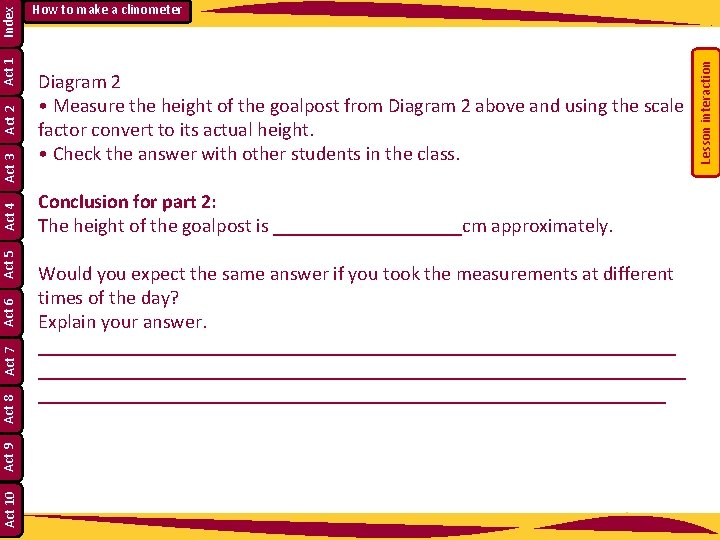Diagram 2 • Measure the height of the goalpost from Diagram 2 above and using the scale factor convert to its actual height. • Check the answer with other students in the class. Conclusion for part 2: The height of the goalpost is __________cm approximately. Would you expect the same answer if you took the measurements at different times of the day? Explain your answer. _________________________________________________________________ Lesson interaction Index Act 1 Act 2 Act 3 Act 4 Act 5 Act 6 Act 7 Act 8 Act 9 Act 10 How to make a clinometer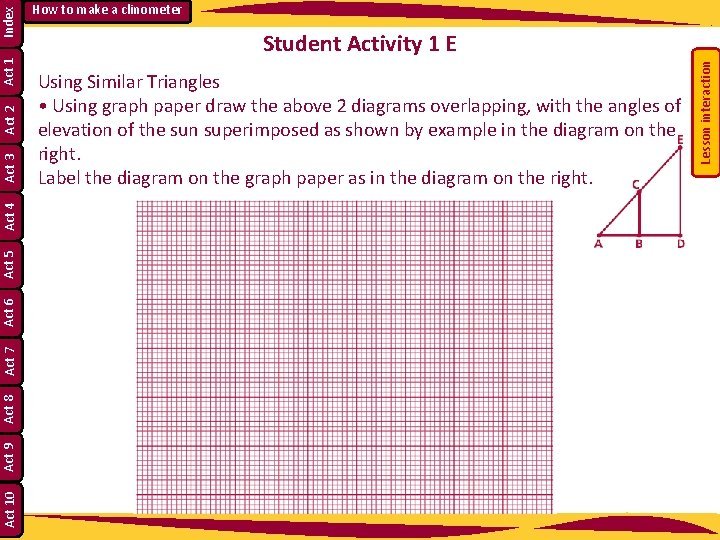Student Activity 1 E Using Similar Triangles • Using graph paper draw the above 2 diagrams overlapping, with the angles of elevation of the sun superimposed as shown by example in the diagram on the right. Label the diagram on the graph paper as in the diagram on the right. Lesson interaction Index Act 1 Act 2 Act 3 Act 4 Act 5 Act 6 Act 7 Act 8 Act 9 Act 10 How to make a clinometer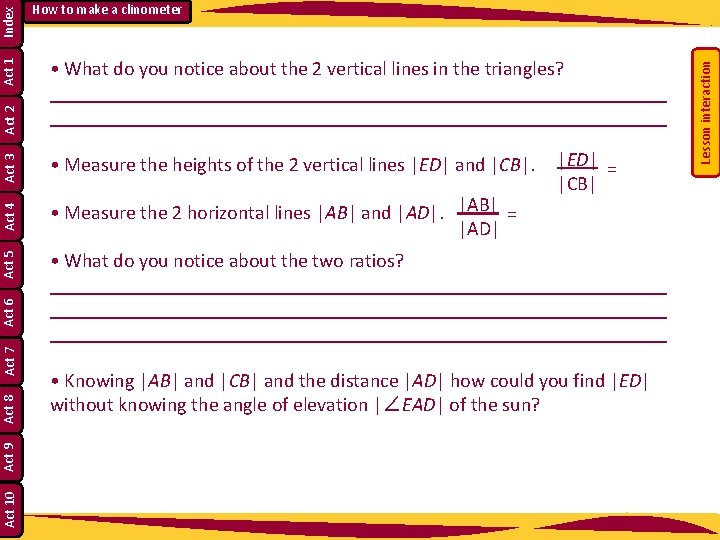• Measure the 2 horizontal lines |AB| and |AD|. |AB| = |AD| Act 5 Act 6 Act 7 Act 8 Act 9 Act 10 |ED| = |CB| • What do you notice about the two ratios? ______________________________________________________________ • Knowing |AB| and |CB| and the distance |AD| how could you find |ED| without knowing the angle of elevation |∠EAD| of the sun? Lesson interaction Index Act 3 • Measure the heights of the 2 vertical lines |ED| and |CB|. Act 1 Act 2 • What do you notice about the 2 vertical lines in the triangles? ______________________________________________________________ Act 4 How to make a clinometerRatios in Similar Triangles • Draw 3 different right angled triangles with the arms of the 90 degree angle being vertical and horizontal line segments, using the same angle of elevation which you calculated for the sun. Call the triangles T 1, T 2, T 3. Lesson interaction Index Act 1 Act 2 Act 3 Act 4 Act 5 Act 6 Act 7 Act 8 Act 9 Act 10 How to make a clinometer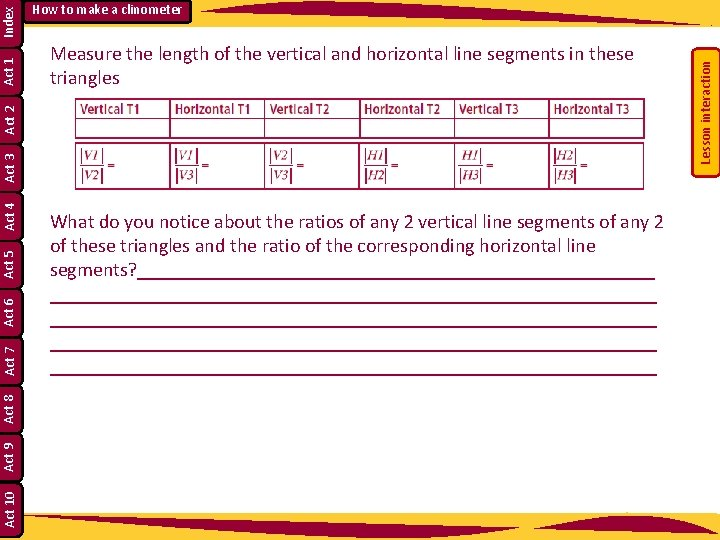Index Act 3 Act 4 Act 5 Act 6 Act 7 Act 8 Act 9 Act 10 What do you notice about the ratios of any 2 vertical line segments of any 2 of these triangles and the ratio of the corresponding horizontal line segments? _____________________________________________________________ _______________________________ Lesson interaction Measure the length of the vertical and horizontal line segments in these triangles Act 2 Act 1 How to make a clinometerStudent Activity 2 Labelling Sides in Right Angled Triangles What is the hypotenuse of a right-angled triangle? Mark the hypotenuse on the triangles in Student Activity 2 of right-angled triangles. How many other angles are in the triangle? How many degrees do they add up to? What are these angles called? Lesson interaction Index Act 1 Act 2 Act 3 Act 4 Act 5 Act 6 Act 7 Act 8 Act 9 Act 10 How to make a clinometerMark either one of the two complementary angles on the triangle with an arc. What is the name of one of the arms of that angle? Label the third side of the triangle as the opposite side. Describe the opposite side. Act 10 Act 9 Act 7 The other side, which is beside the marked angle, is given the name “adjacent”. Label it. Lesson interaction Labelling Sides in Right Angled Triangles Lesson interaction Index Act 1 Act 2 Act 3 Act 4 Act 5 Student Activity 2 Act 8 Act 6 How to make a clinometerAct 4 When giving the ratios use the names for the sides. Opp Hyp Adj Hyp Opp Hyp Adj Opp What is the relationship between the first pair? Act 10 Act 9 Act 7 How many possible ratios could be worked out for a right angled triangle? Act 8 Would the order matter? Explain your answer. Act 5 If I knew the answer to the first ratio was ½, what would be the answer to the second one? Lesson interaction If you were to work out the ratio of any two of the sides in a right angled triangle how would you do this? Lesson interaction Index Act 1 Act 2 Repeat this process of marking angles and labelling sides for all the triangles on Student Activity 1. Act 6 Act 3 How to make a clinometerFor each triangle of the five triangles mark the 90° angle and one other given angle (given on the sheet). Label the sides of the right angled triangles as hyp (hypotenuse), adj (adjacent), and opp (opposite). Measure each side correct to the nearest mm and calculate the ratios opp/hyp, adj/hyp, opp/adj. One student is to measure, one to calculate ratios and one to double check changing roles on each triangle. Lesson interaction Student Activities 3, 4, 5, 6, 7 and 8: Lesson interaction Index Act 1 Act 2 Act 3 Act 4 Act 5 Act 6 Act 7 Act 8 Act 9 Act 10 How to make a clinometer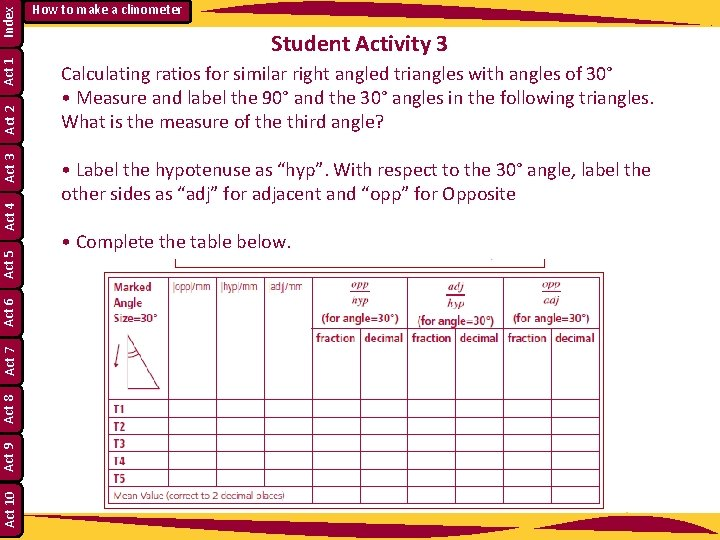Index Act 1 Act 2 Act 3 Act 4 Act 5 Act 6 Act 7 Act 8 Act 9 Act 10 How to make a clinometer Student Activity 3 Calculating ratios for similar right angled triangles with angles of 30° • Measure and label the 90° and the 30° angles in the following triangles. What is the measure of the third angle? • Label the hypotenuse as “hyp”. With respect to the 30° angle, label the other sides as “adj” for adjacent and “opp” for Opposite • Complete the table below.Index Act 1 Act 2 Act 3 Act 4 Act 5 Act 6 Act 7 Act 8 Act 9 Act 10 How to make a clinometer Student Activity 4 Calculating ratios for similar right angled triangles with angles of 40° • Measure and label the 90° and the 40° angles in the following triangles. What is the measure of the third angle? • Label the hypotenuse as “hyp”. With respect to the 40° angle, label the other sides as “adj” for adjacent and “opp” for opposite. • Complete the table below.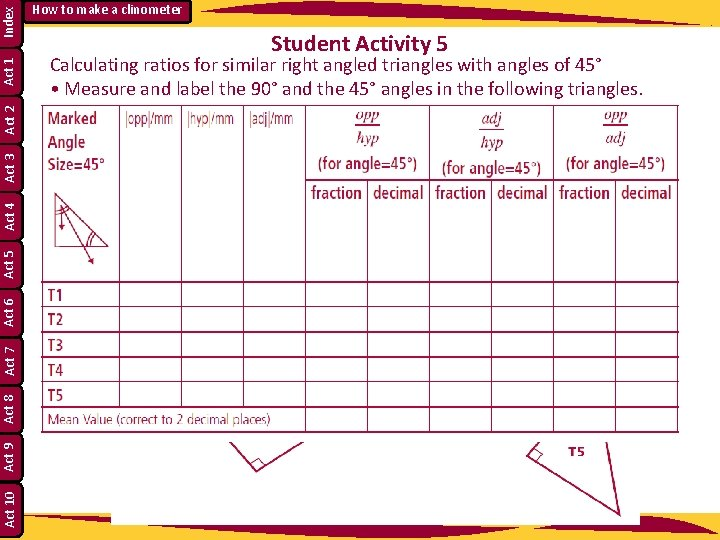Index Act 1 Act 2 Act 3 Act 4 Act 5 Act 6 Act 7 Act 8 Act 9 Act 10 How to make a clinometer Student Activity 5 Calculating ratios for similar right angled triangles with angles of 45° • Measure and label the 90° and the 45° angles in the following triangles. What types of right angled triangle are these triangles? _____________ • Label the hypotenuse as “hyp”. With respect to the 45° angle, label the other sides as “adj” for adjacent and “opp” for opposite. • Complete the table below.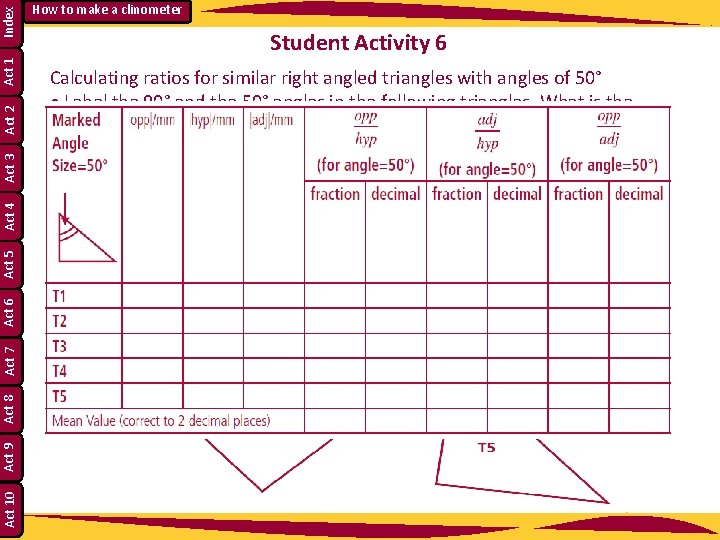Index Act 1 Act 2 Act 3 Act 4 Act 5 Act 6 Act 7 Act 8 Act 9 Act 10 How to make a clinometer Student Activity 6 Calculating ratios for similar right angled triangles with angles of 50° • Label the 90° and the 50° angles in the following triangles. What is the measure of the third angle? _________________________ • Label the hypotenuse as “hyp”. With respect to the 50° angle, label the other sides as “adj” for adjacent and “opp” for opposite. • Complete the table below.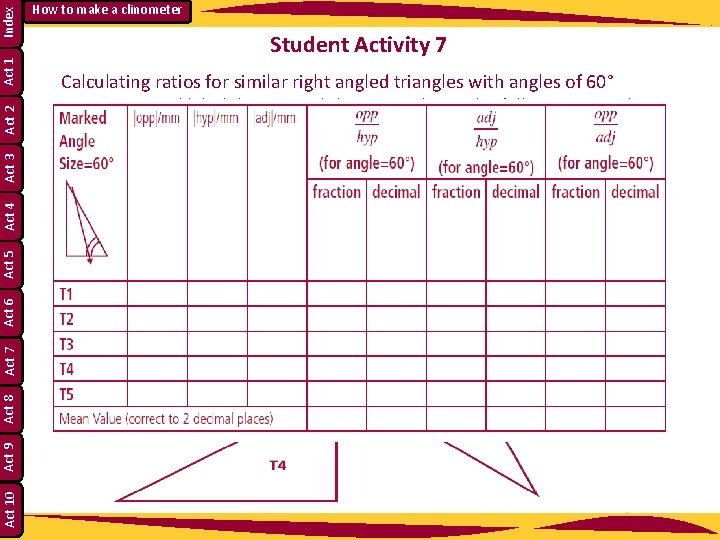Index Act 1 Act 2 Act 3 Act 4 Act 5 Act 6 Act 7 Act 8 Act 9 Act 10 How to make a clinometer Student Activity 7 Calculating ratios for similar right angled triangles with angles of 60° • Measure and label the 90° and the 60° angles in the following triangles. What is the measure of the third angle? ____________________ • Label the hypotenuse as “hyp”. With respect to the 60° angle, label the other sides as “adj” for adjacent and “opp” for opposite. • Complete the table below.Index Act 1 Act 2 Act 3 Act 4 Act 5 Act 6 Act 7 Act 8 Act 9 Act 10 How to make a clinometer Student Activity 8 Calculating ratios for similar right angled triangles with angles of 70° • Measure and label the 90° and the 70° angles in the following triangles. What is the measure of the third angle? ______________ • Label the hypotenuse as “hyp”. With respect to the 70° angle, label the other sides as “adj” for adjacent and “opp” for opposite. • Complete the table below.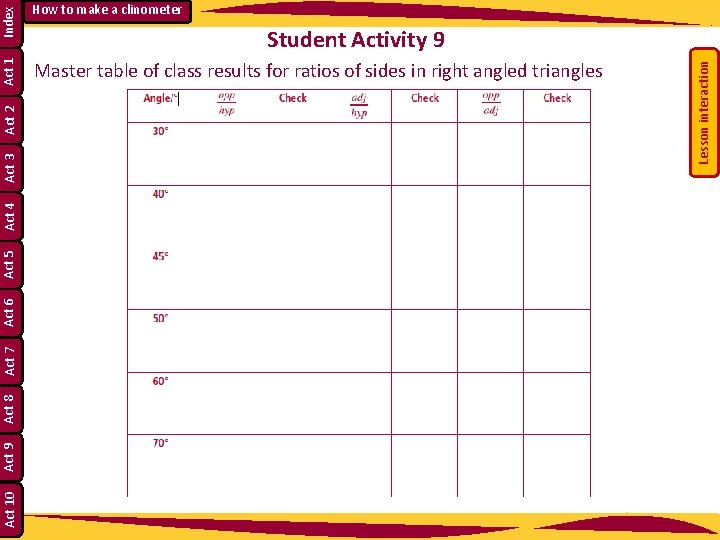Index Act 1 Master table of class results for ratios of sides in right angled triangles Act 10 Act 9 Act 8 Act 7 Act 6 Act 5 Act 4 Student Activity 9 Lesson interaction Act 3 Act 2 How to make a clinometerStudent Activity 10 Using the master table of class results answer the following questions 1. What do you notice about sin 30° and cos 60°? ______________________________________________ 2. What do you notice about cos 30° and sin 60°? ______________________________________________ 3. Can you explain what you have noticed using diagrams? 4. How would you describe angles 30° and 60°? _______________________________________________ 5. Can you find similar examples in the master table? __________________________________________________________________ 6. For what angle in a right angled triangle is the opposite side one half of the hypotenuse? ____________________________________________________________ Draw a diagram to illustrate your answer. 7. For what angle in a right angled triangle are the opposite and adjacent sides equal? ________________________________ Lesson interaction Index Act 1 Act 2 Act 3 Act 4 Act 5 Act 6 Act 7 Act 8 Act 9 Act 10 How to make a clinometer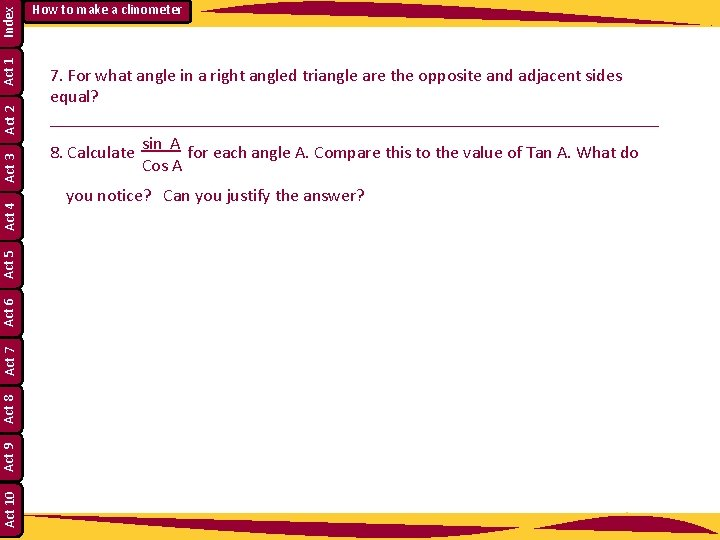Index Act 1 Act 2 Act 3 Act 4 Act 5 Act 6 Act 7 Act 8 Act 9 Act 10 How to make a clinometer 7. For what angle in a right angled triangle are the opposite and adjacent sides equal? __________________________________ 8. Calculate sin A for each angle A. Compare this to the value of Tan A. What do Cos A you notice? Can you justify the answer?Index Act 1 Act 2 Act 3 Act 4 Act 5 Act 6 Act 7 Act 8 Act 9 Act 10 How to make a clinometer Making and using a clinometer to find the height of a tall structure Materials required: Protractor, sellotape, drinking straw, needle and thread, paper clip.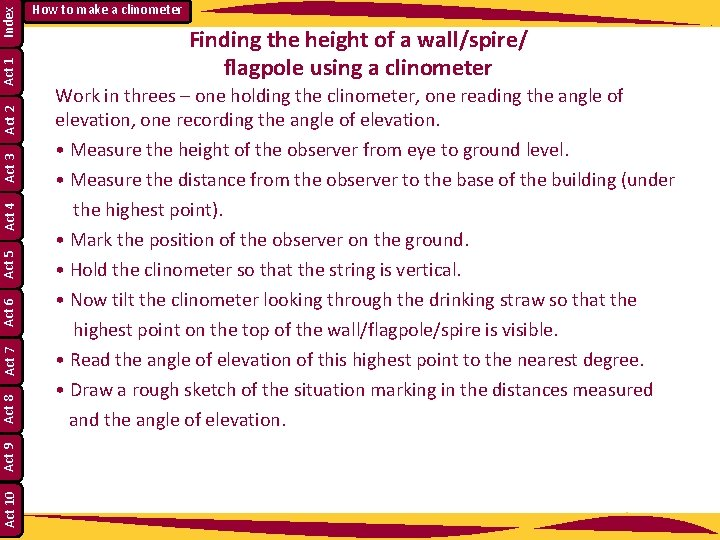Index Act 1 Act 2 Act 3 Act 4 Act 5 Act 6 Act 7 Act 8 Act 9 Act 10 How to make a clinometer Finding the height of a wall/spire/ flagpole using a clinometer Work in threes – one holding the clinometer, one reading the angle of elevation, one recording the angle of elevation. • Measure the height of the observer from eye to ground level. • Measure the distance from the observer to the base of the building (under the highest point). • Mark the position of the observer on the ground. • Hold the clinometer so that the string is vertical. • Now tilt the clinometer looking through the drinking straw so that the highest point on the top of the wall/flagpole/spire is visible. • Read the angle of elevation of this highest point to the nearest degree. • Draw a rough sketch of the situation marking in the distances measured and the angle of elevation.